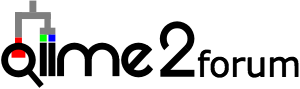# When to recalculate RPCA

Hi @cmartino, @mortonjt, and hopefully@fedarko (and sorry if Im wrong),

This is probably a super basic DECOIDE question, but is the underlying Aitchinson distance matrix stable? I’m assuming that if I filter out a sample from the distance matrix, it shouldn’t/won’t affect the other distances? However, I’m also assuming that I need to re-calculate the PCA portion if my underlying sequences change?

Thank you all for the sanity check!

3 Likes

Hi @jwdebelius,

This is a great question! In RPCA (DEICODE) the distances are calculated from the dimentionality reduction loadings (opposite to beta-diveristy/PCoA). This means the distance matrix will change if you remove samples in RPCA (more or less depending on the sample). This would not be true in any other beta-diveristy method because the distances are calculated pairwise before PCoA. Conventional Aitchison PCA distance will also not change after sample add/dropout because the distance matrix is made first, which is just the Eucledian distance on a CLR transformed table (using psuedocount).